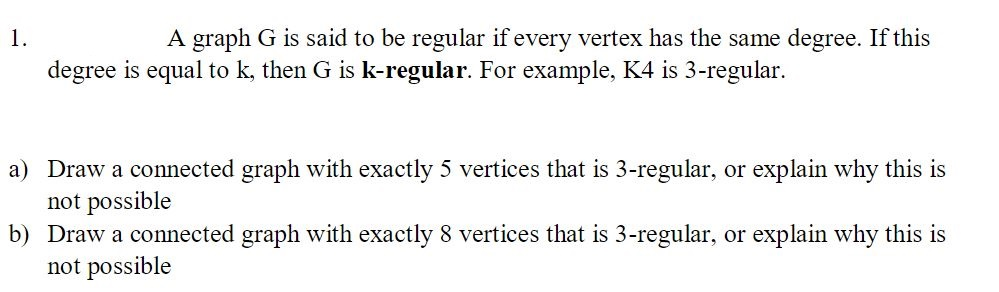# A graph G is said to be regular if every vertex has the same degree. If this1degree is equal to k, then G is k-regular. For example, K4 is 3-regulara) Draw a connected graph with exactly 5 vertices that is 3-regular, or explain why this isnot possibleb) Draw a connected graph with exactly 8 vertices that is 3-regular, or explain why this isnot possible

Question
55 viewshelp_outlineImage TranscriptioncloseA graph G is said to be regular if every vertex has the same degree. If this 1 degree is equal to k, then G is k-regular. For example, K4 is 3-regular a) Draw a connected graph with exactly 5 vertices that is 3-regular, or explain why this is not possible b) Draw a connected graph with exactly 8 vertices that is 3-regular, or explain why this is not possible fullscreen
check_circle

Step 1

K-regular graph means each vertex has k degree.

Step 2

If Graph, H is 3- regular then degree of each vertex in H is 3.

Number of vertices in H is 5.

So, sum of degrees of all vertices will be,

Step 3

The number of edges is half of the sum of the degrees of...

### Want to see the full answer?

See Solution

#### Want to see this answer and more?

Solutions are written by subject experts who are available 24/7. Questions are typically answered within 1 hour.*

See Solution
*Response times may vary by subject and question.
Tagged in

### Math